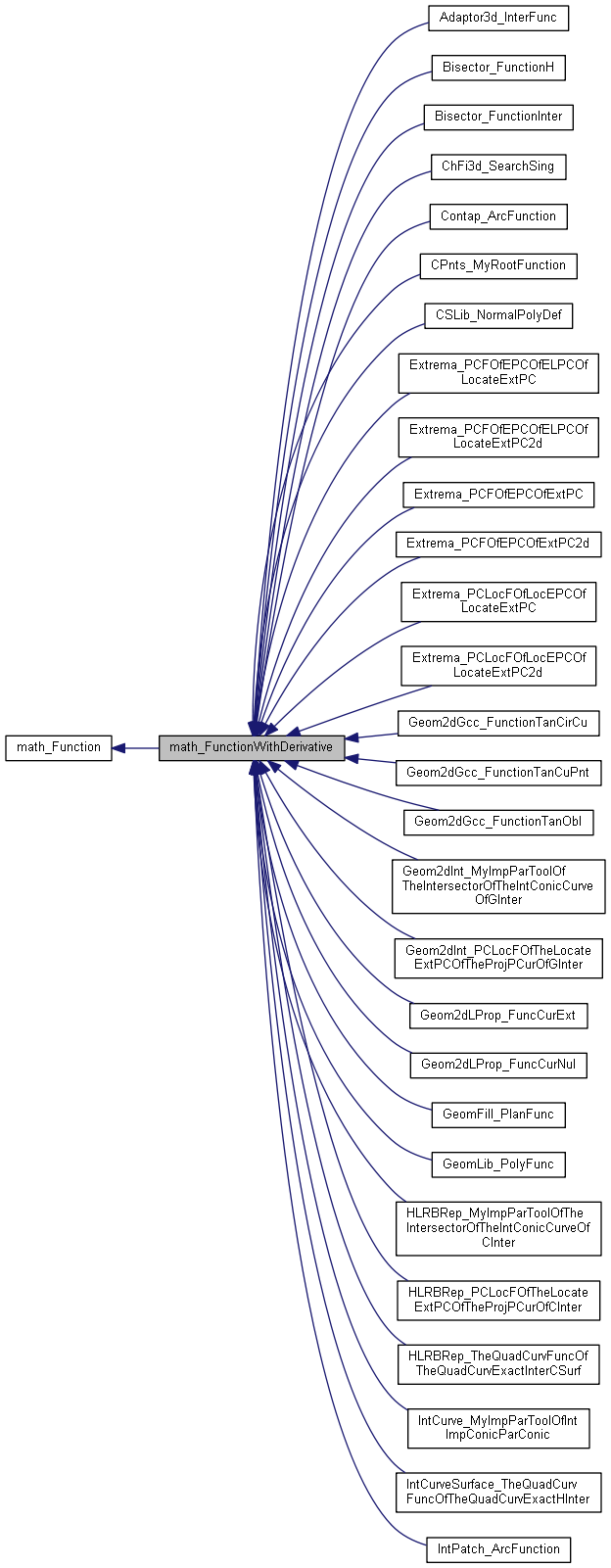# math_FunctionWithDerivative Class Referenceabstract

This abstract class describes the virtual functions associated with a function of a single variable for which the first derivative is available. More...

`#include <math_FunctionWithDerivative.hxx>`

Inheritance diagram for math_FunctionWithDerivative:[legend]

## Public Member Functions

virtual Standard_Boolean Value (const Standard_Real X, Standard_Real &F)=0
Computes the value <F>of the function for the variable <X>. Returns True if the calculation were successfully done, False otherwise. More...

virtual Standard_Boolean Derivative (const Standard_Real X, Standard_Real &D)=0
Computes the derivative <D> of the function for the variable <X>. Returns True if the calculation were successfully done, False otherwise. More...

virtual Standard_Boolean Values (const Standard_Real X, Standard_Real &F, Standard_Real &D)=0
Computes the value <F> and the derivative <D> of the function for the variable <X>. Returns True if the calculation were successfully done, False otherwise. More...

virtual ~math_FunctionWithDerivative ()Public Member Functions inherited from math_Function
virtual ~math_Function ()
Virtual destructor, for safe inheritance. More...

virtual Standard_Integer GetStateNumber ()
returns the state of the function corresponding to the latest call of any methods associated with the function. This function is called by each of the algorithms described later which defined the function Integer Algorithm::StateNumber(). The algorithm has the responsibility to call this function when it has found a solution (i.e. a root or a minimum) and has to maintain the association between the solution found and this StateNumber. Byu default, this method returns 0 (which means for the algorithm: no state has been saved). It is the responsibility of the programmer to decide if he needs to save the current state of the function and to return an Integer that allows retrieval of the state. More...

## Detailed Description

This abstract class describes the virtual functions associated with a function of a single variable for which the first derivative is available.

## Constructor & Destructor Documentation

 virtual math_FunctionWithDerivative::~math_FunctionWithDerivative ( )
virtual

## Member Function Documentation

 virtual Standard_Boolean math_FunctionWithDerivative::Derivative ( const Standard_Real X, Standard_Real & D )
pure virtual
 virtual Standard_Boolean math_FunctionWithDerivative::Value ( const Standard_Real X, Standard_Real & F )
pure virtual
 virtual Standard_Boolean math_FunctionWithDerivative::Values ( const Standard_Real X, Standard_Real & F, Standard_Real & D )
pure virtual

Computes the value <F> and the derivative <D> of the function for the variable <X>. Returns True if the calculation were successfully done, False otherwise.

The documentation for this class was generated from the following file: